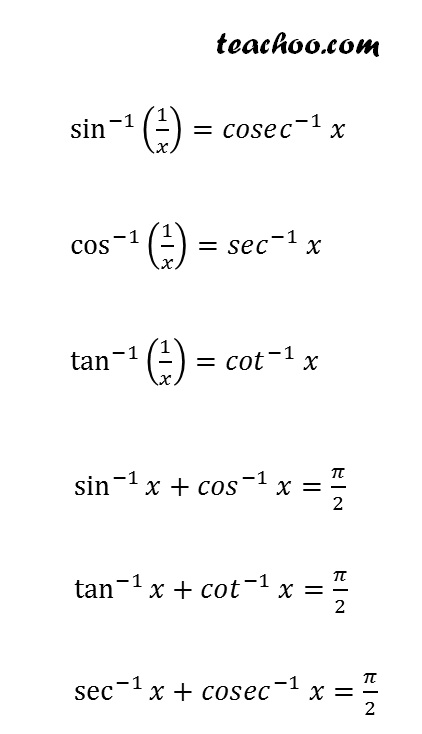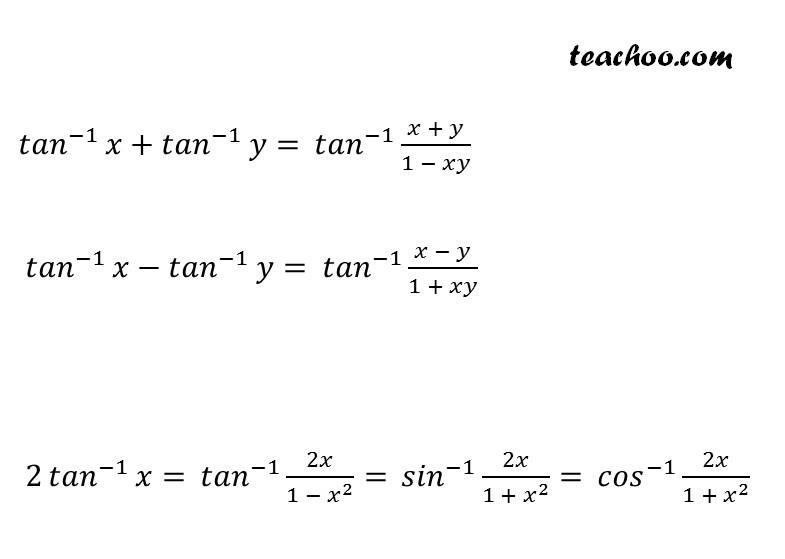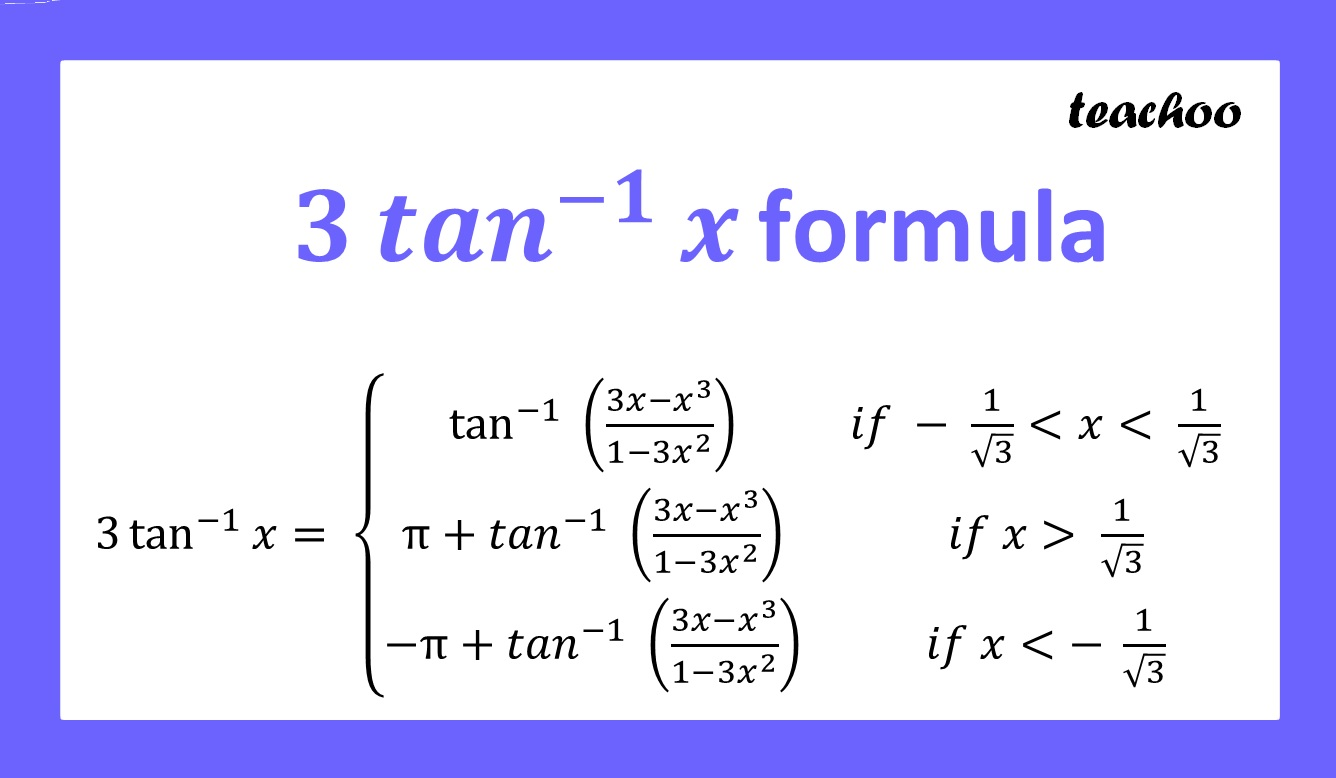Formulae based

Chapter 2 Class 12 Inverse Trigonometric Functions
Concept wise

Some formulae for Inverse Trigonometry are

sin –1 (–x) = – sin -1 x

cos –1 (–x) = π – cos  -1 x

tan –1 (–x) = – tan -1 x

cosec –1 (–x) = – cosec -1 x

sec –1 (–x) = π – sec -1 x

cot –1 (–x) = π – cot -1 xLearn in your speed, with individual attention - Teachoo Maths 1-on-1 Class

### Transcript

3 tan^(−1)⁡𝑥 "formula" 3 tan^(−1)⁡𝑥= {■8(tan^(−1) ((3𝑥−𝑥^3)/(1−3𝑥^2 ))&𝑖𝑓 − 1/√3<𝑥< 1/√3 @〖π+𝑡𝑎𝑛〗^(−1) ((3𝑥−𝑥^3)/(1−3𝑥^2 ))&𝑖𝑓 𝑥> 1/√3@〖−π+𝑡𝑎𝑛〗^(−1) ((3𝑥−𝑥^3)/(1−3𝑥^2 ))&𝑖𝑓 𝑥<− 1/√3)┤